Courses

# Ideal Versus Practical Resistance Levels Electrical Engineering (EE) Notes | EduRev

## Electronic Devices

Created by: Cstoppers Instructors

## Electrical Engineering (EE) : Ideal Versus Practical Resistance Levels Electrical Engineering (EE) Notes | EduRev

The document Ideal Versus Practical Resistance Levels Electrical Engineering (EE) Notes | EduRev is a part of the Electrical Engineering (EE) Course Electronic Devices.
All you need of Electrical Engineering (EE) at this link: Electrical Engineering (EE)

IDEAL VERSUS PRACTICAL RESISTANCE LEVELS

DC or Static Resistance

The application of a dc voltage to a circuit containing a semiconductor diode will result in an operating point on the characteristic curve that will not change with time. The resistance of the diode at the operating point can be found simply by finding the corresponding levels of VD and ID as shown in Fig. 1.25 and applying the following equation: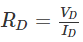The dc resistance levels at the knee and below will be greater than the resistance levels obtained for the vertical rise section of the characteristics. The resistance levels in the reverse-bias region will naturally be quite high. Since ohmmeters typically employ a relatively constant-current source, the resistance determined will be at a preset current level (typically, a few milliamperes).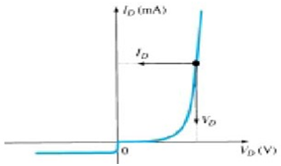Figure 1.25 To determine the dc resistance of a diode at a particular operating point.

AC or Dynamic Resistance

It is obvious from Eq. 1.5 that the dc resistance of a diode is independent of the shape of the characteristic in the region surrounding the point of interest. If a sinusoidal input (rather than dc input) is applied, the situation will change completely. The varying input will move the instantaneous operating point up and down the region of the characteristics, thus defining a specific change in current and voltage as shown in Fig. 1.27. If the applied signal is not varying in nature, the point of operation would be the Q-point appearing on Fig. 1.27 determined by the applied dc levels. The designation Q-point is derived from the word quiescent, which means “still or unvarying.”  A straight-line drawn tangent to the curve through the Q-point as shown in Fig. 1.28 will define a particular change in voltage and current that can be used to determine the ac or dynamic resistance for this region of the diode characteristics. In equation form,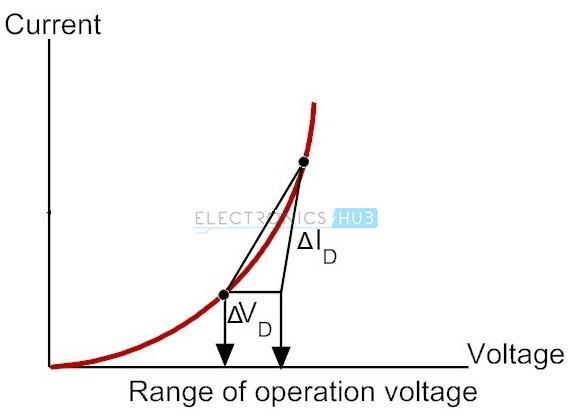Fig. 1.27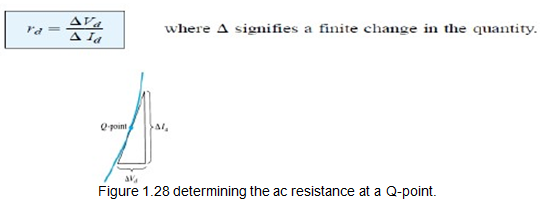DIODE EQUIVALENT CIRCUITS

An equivalent circuit is a combination of elements properly chosen to best represent the actual terminal characteristics of a device, system, or in a particular operating region. In other words, once the equivalent circuit is defined, the device symbol can be removed from a schematic and the equivalent circuit inserted in its place without severely affecting the actual behaviour of the system. The result is often a network that can be solved using  the traditional circuit analysis techniques.

Piecewise-Linear Equivalent Circuit

One technique for obtaining an equivalent circuit for a diode is to approximate the characteristics of the device by straight-line segments, as shown in Fig. 1.31. The resulting equivalent circuit is naturally called the piecewise-linear equivalent circuit. It should be obvious from Fig. 1.31 that the straight-line segments do not result in an exact duplication of the actual characteristics, especially in the knee region. However, the resulting segments are sufficiently close to the actual curve to establish an equivalent circuit that will provide an excellent first approximation to the actual behavior of the device. The ideal diode is included to establish that there is only one direction of conduction through the device, and a reverse-bias condition will result in the open- circuit state for the device. Since a silicon semiconductor diode does not reach the conduction state until VD reaches 0.7 V with a forward bias (as shown in Fig. 1.31), a battery VT opposing the conduction direction must appear in the equivalent circuit as shown in Fig. 1.32. The battery simply specifies that the voltage across the device must be greater than the threshold battery voltage before conduction through the device, in the direction dictated by the ideal diode can be established. When conduction is established, the resistance of the diode will be the specified value of rav.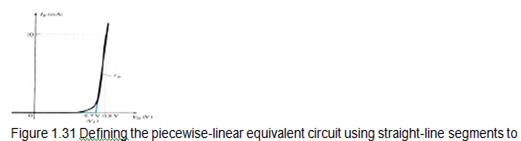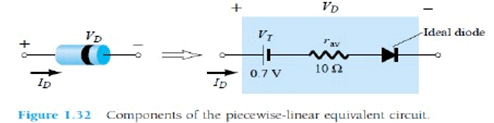The approximate level of rav can usually be determined from a specified operating point on the specification sheet. For instance, for a silicon semiconductor diode, if I=10 mA (a forward conduction current for the diode) at V=0.8 V, we know for silicon that a shift of 0.7 V is required before the characteristics rise.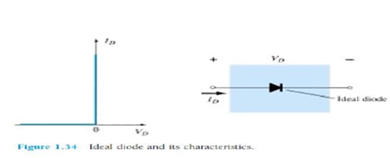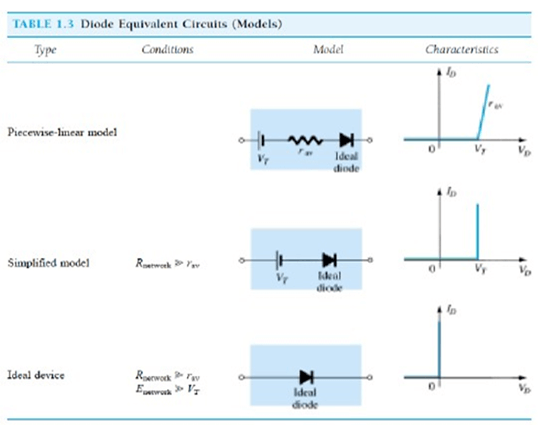Offer running on EduRev: Apply code STAYHOME200 to get INR 200 off on our premium plan EduRev Infinity!

,

,

,

,

,

,

,

,

,

,

,

,

,

,

,

,

,

,

,

,

,

;